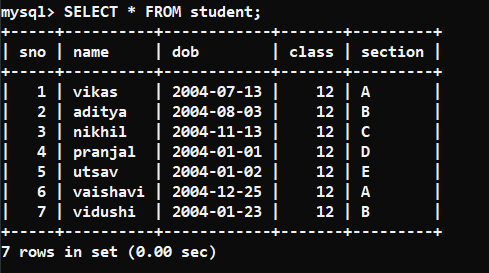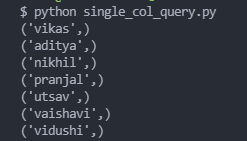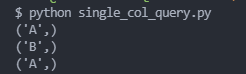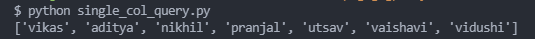# Single column query results in SQLAlchemy

• Last Updated : 28 Feb, 2022

In this post, we are going to deep dive into the results obtained when we run a single-column query using SQLAlchemy.

SQLAlchemy is an awesome and easy-to-use python module that is used to connect python and SQL databases together increasing the powers of any programmer. To install SQLAlchemy, run the following command in your terminal:

`pip install sqlalchemy pymysql`

Note: pymysql is a dependency of SQLAlchemy which we need to install for this post.

So, when we run a single column query using SQLAlchemy we get a result. The result object will contain tuples of values of that single column query. This is how the basic functionality works.

Let us run some queries and see the above

Database used:### Example 1:

Let us fetch all the names using SQLAlchemy and see the result obtained. The SQL query will look like this:

`SELECT name FROM student;`

## Python3

 `from` `sqlalchemy ``import` `create_engine ` ` `  `user, password, host, database ``=` `'root'``, ``'123'``, ``'localhost'``, ``'geeksforgeeks'` `engine ``=` `create_engine( ` `    ``url``=``f``'mysql+pymysql://{user}:{password}@{host}/{database}?charset=utf8'``) ` ` `  `connection ``=` `engine.connect() ` ` `  `table_name ``=` `'student'` ` `  `query ``=` `f``'SELECT name FROM {table_name} ;'` `result ``=` `connection.execute(query) ` ` `  `for` `e ``in` `result: ` `    ``print``(e) `Output

### Example 2:

Let us fetch all the sections of students with sno is 1 or 2 or 6 from the student’s table.

The SQL query will look like this:

`SELECT section FROM student WHERE sno IN (1,2,6);`

## Python3

 `from` `sqlalchemy ``import` `create_engine ` ` `  `user, password, host, database ``=` `'root'``, ``'123'``, ``'localhost'``, ``'geeksforgeeks'` `engine ``=` `create_engine( ` `    ``url``=``f``'mysql+pymysql://{user}:{password}@{host}/{database}?charset=utf8'``) ` ` `  `connection ``=` `engine.connect() ` ` `  `table_name ``=` `'student'` ` `  `query ``=` `f``'SELECT section FROM {table_name} WHERE sno IN (1,2,6) ;'` `result ``=` `connection.execute(query) ` ` `  `for` `e ``in` `result: ` `    ``print``(e) `Output

So, as you can see in both of the above examples, the single-column query returns the result which contains tuples whose elements are values fetched from the query.

## Getting a list that contains direct values of results in place of tuples

Now, you might require that you can get the values directly in place of being stored in a tuple and getting those tuples.

As you can observe, the first element of each tuple is our value. So, you can run a for loop on the result object and in each iteration, you will get the tuple. And you can append the first element of that tuple to your custom list and easily get those values. Let us see the code for the above:

## Python3

 `values ``=` `[] ` `for` `e ``in` `result: ` `    ``values.append(e[``0``])`

The values list will contain all the fetched values directly. You can reduce the no. of lines used above by using list comprehension. The code for that is :

## Python3

 `values ``=` `[ e[``0``] ``for` `e ``in` `result ] `

## Python3

 `from` `sqlalchemy ``import` `create_engine ` ` `  `user, password, host, database ``=` `'root'``, ``'123'``, ``'localhost'``, ``'geeksforgeeks'` `engine ``=` `create_engine( ` `    ``url``=``f``'mysql+pymysql://{user}:{password}@{host}/{database}?charset=utf8'``) ` ` `  `connection ``=` `engine.connect() ` ` `  `table_name ``=` `'student'` ` `  `query ``=` `f``'SELECT name FROM {table_name};'` `result ``=` `connection.execute(query) ` ` `  `values ``=` `[] ` `for` `e ``in` `result: ` `    ``values.append(e[``0``]) ` ` `  `print``(values) `Output

My Personal Notes arrow_drop_up
Recommended Articles
Page :